# 男士polo衫T恤的比例法制图

34599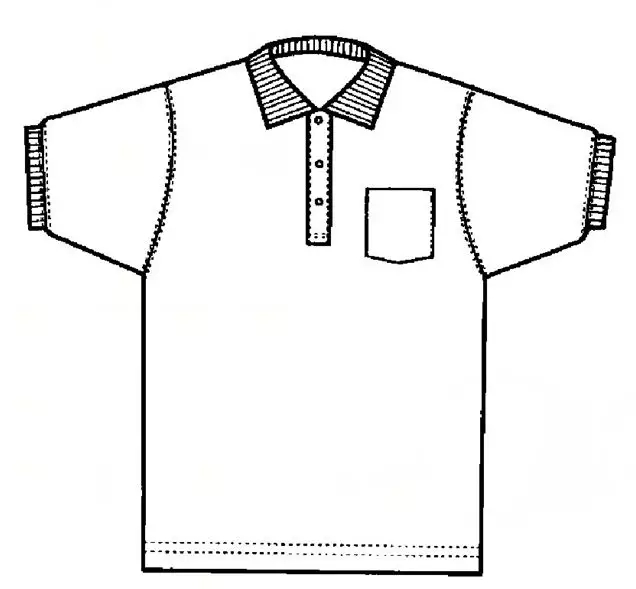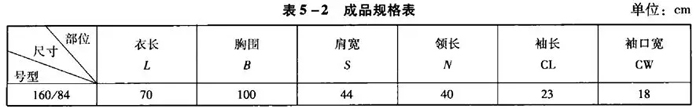(1)前片

①作基础线：先画上平线，然后垂直于上平线画一条直线作为前中线。

②衣长：衣长+自然回缩=70cm×(1+自然回缩率)=70cm×(1+2%)=71.4cm。

③领宽：N/5=40cm/5=8cm。

④领深：N/5+0.5cm=40cm/5+0.5cm=8.5cm。

⑤落肩：S/10=44cm/10=4.4。

⑥肩宽：S/2=44cm/2=22cm。

⑦油底深：胸围/6+6cm=100cm/6+6cm=22.5cm(小数只保留0.5cm，如不足0.5cm舍去)。

⑧胸围宽：B/4=100cm/4=25cm。

(2)后片

①延长上平线、底边线。

②领宽：同前片。

③领深：1.5cm。

④落肩：同前片。

⑤肩宽：同前片。

⑥柚糜深：同前片。

⑦胸围宽：同前片。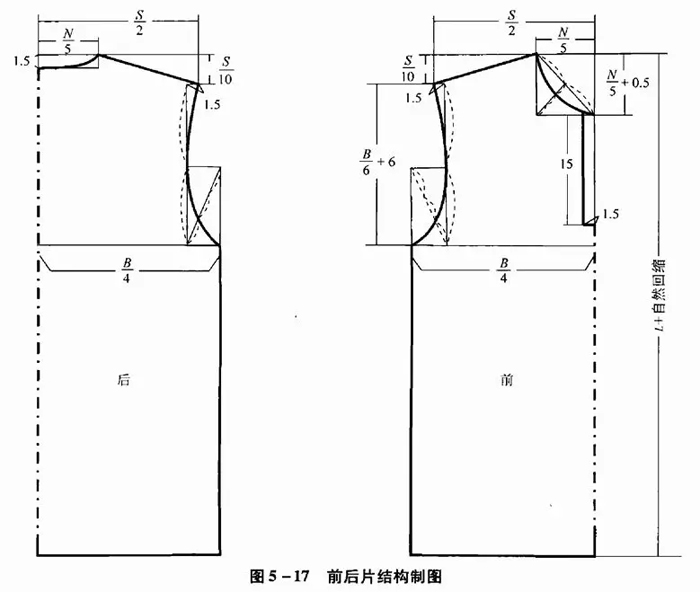(3)袖片

①袖长：袖长+自然回缩=(23cm-2.5cm)×(1+1%)=23.2cm。

②袖山高：B/10=100cm/10=10cm。

③柚口宽：20.5cm。

④罗纹袖口宽：18cm。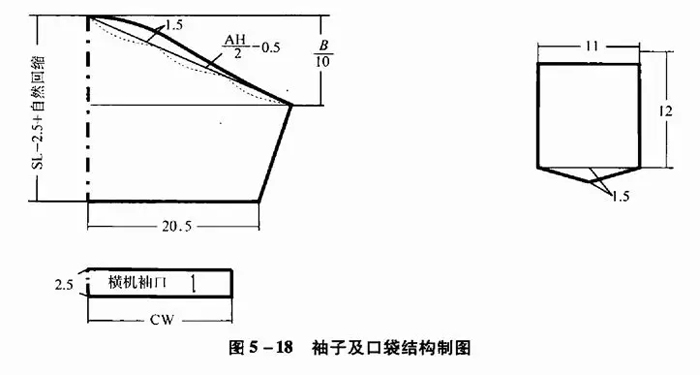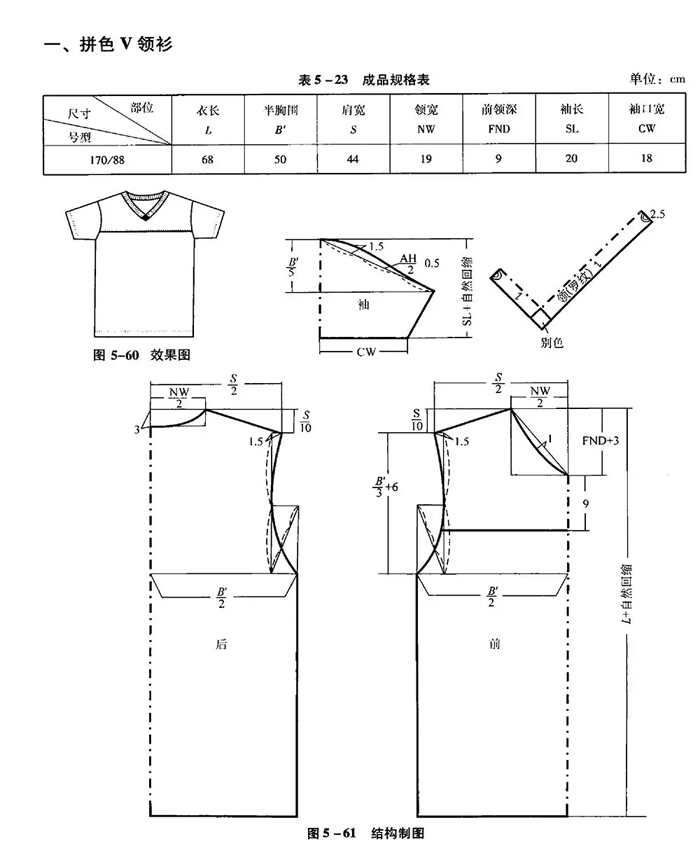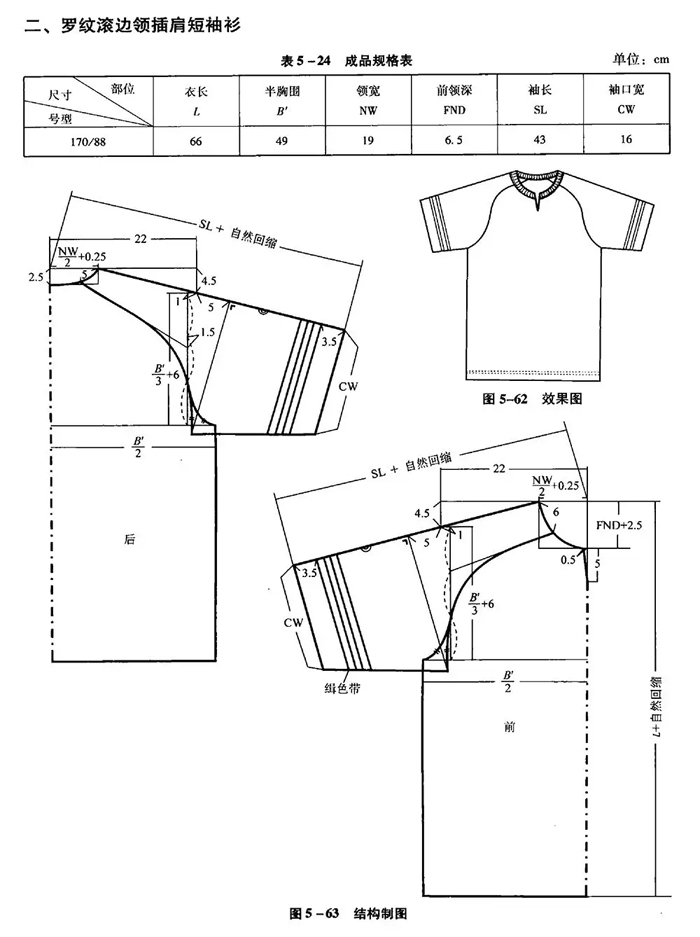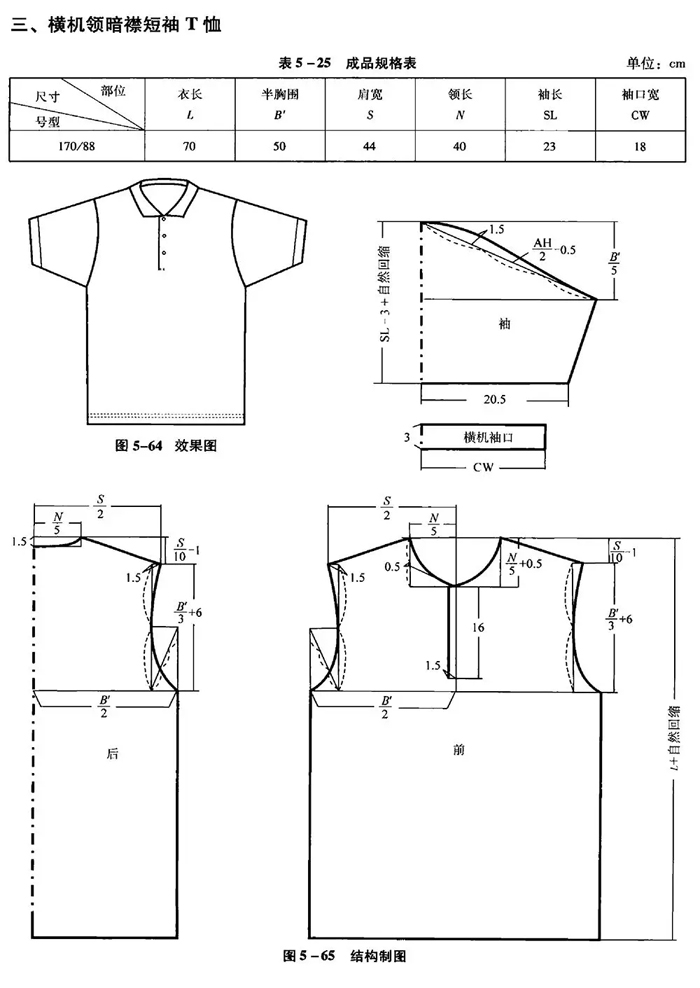9